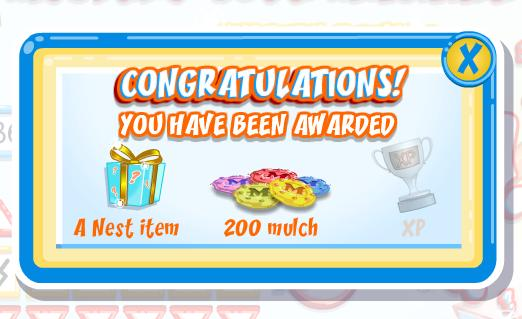# December 18 Weekend Puzzle Challenge Cheats

There is a brand new weekend challenge in Bin Weevils, and that means there is a brand new reward to unlock!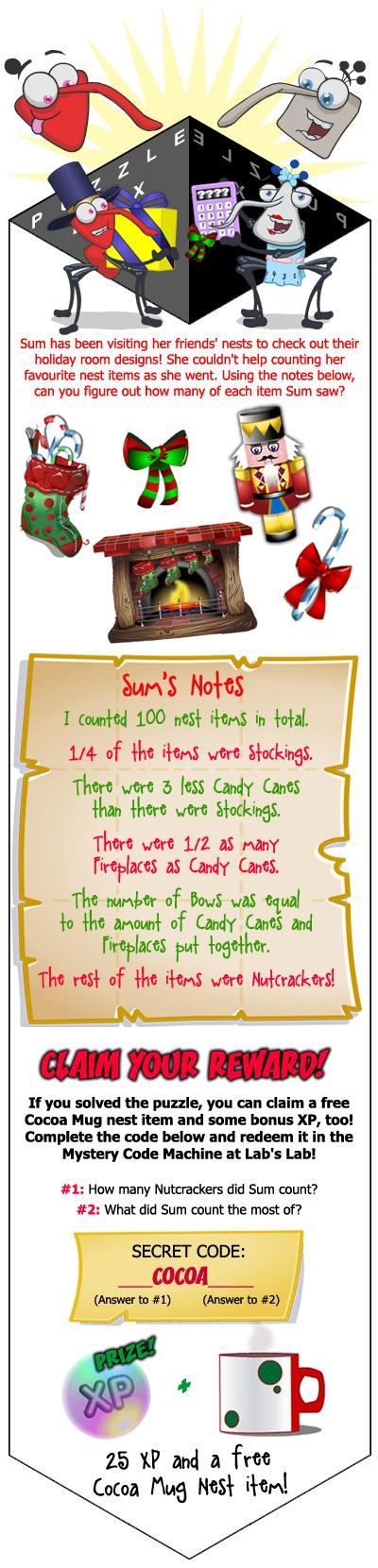Let’s look at the logic behind solving this problem:

• There was a total of 100 items.
• 1/4 of the items were stockings. That means there were 25 stockings.
• There were 3 less candy canes than there were stockings. That means there were 22 candy canes.
• There were 1/2 as many fireplaces as candy canes. That means there were 11 fireplaces.
• The amount of bows was equal to the amount of candy canes and fireplaces put together. There were 22 candy canes and 11 fireplaces, so there was a total of 33 bows.
• The rest of the items are nutcrackers. So far, 91 items have been accounted for. That means the remaining 9 are nutcrackers.

For question 1, there was a total of 9 nutcrackers. For question 2, Sum counted the most of bows (as there was a total of 33). That makes the secret code 9cocoabows!

# Free Christmas Tree at Flem Manor

If you go to Flem Manor, you can get a FREE Christmas tree for your nest!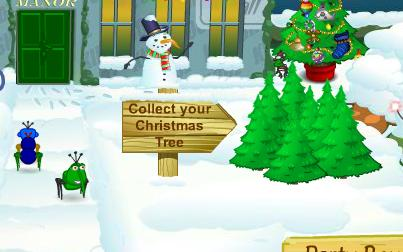Clicking on the Christmas Trees will launch a popup, where you can pick up your free tree (for both tycoons and free users).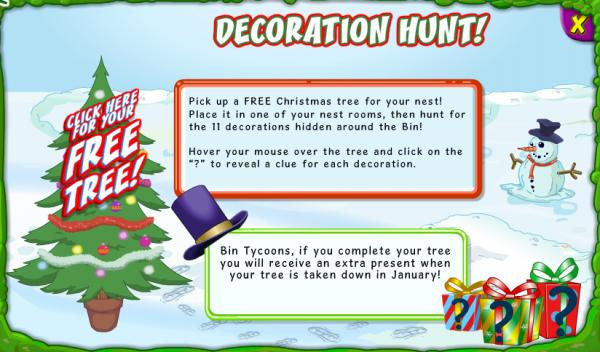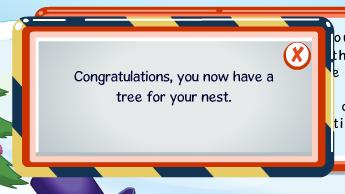Take a look. Looks pretty cool, don’t you think? Did you also get a free tree? Let me know!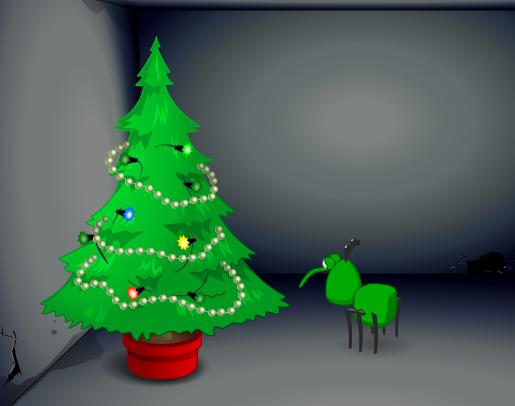# December 11 Weekend Puzzle Challenge Cheats

Clott from Bin Weevils has posted a puzzle challenge on their site. We will provide the solution for the puzzle after the image below.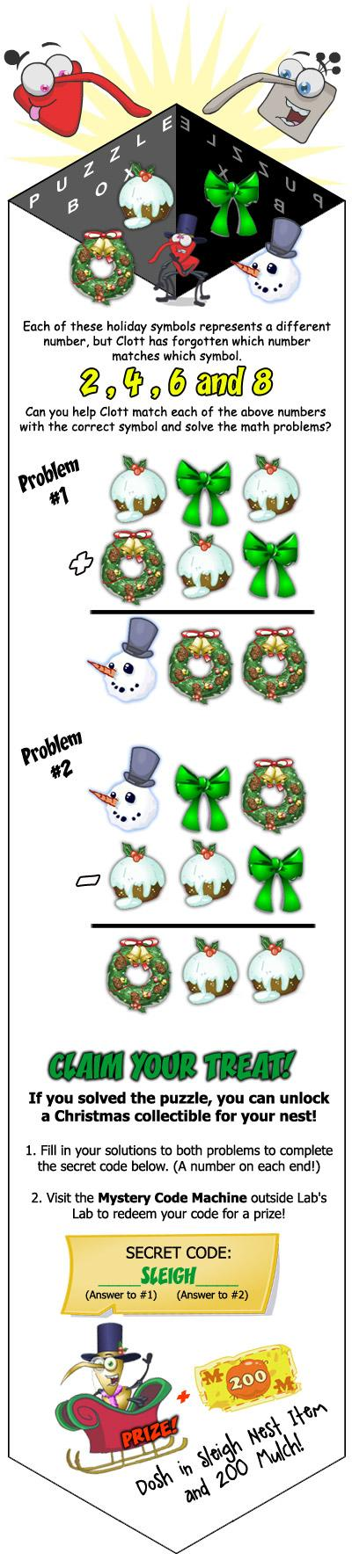As the above image states, each different symbol stands for either 2, 4, 6, or 8. The answer to problem 1 is 866, and the answer to problem 2 is 622. This makes the final code 866sleigh622.

Let’s look at how I deduced the answer to the two problems:

1. We will use C to represent the cupcake, W to represent the wreath, B to represent the bowtie, and S to represent the snowman.
2. When adding two single digit numbers, the highest number you can carry over is 1. You can verify this by adding the two greatest one digit number, which will give you 18 (you will only be able to carry over a 1).
3. We know you won’t need to carry at all in the above math problem. This is because if you have a remainder of 1, you will have an odd number somewhere in the problem. There aren’t any symbols to represent odd numbers, so we know there aren’t any odd numbers.
4. We know none of the addends can contain the number 8. This is because 8 added to the smallest possible other number (2) would require you to carry. As we mentioned previously in step 3, you will not need to carry in this problem.
5. We can conclude S (the snowman) represents the number 8. This is because that’s the only digit that doesn’t appear in any of the addends (similar to what was mentioned in step 4).
6. We know C + W is 8, based on what we deduced in step 5. We know C or W are either 2 or 6. They cannot be 4 because they are two different numbers that sum up to 8.
7. Because neither C or W is 4 as we mentioned in step 6, we know the remaining B is 4.
8. Looking at the ones column, we know C + 4 = W. As mentioned in step 6, C and W are either 2 or 6. In this case, it makes sense to say C is 2 and W is 6.

After you have the code (866sleigh622), go to Lab’s Lab and click on Mystery’s Nest Items. There, you will enter the above code.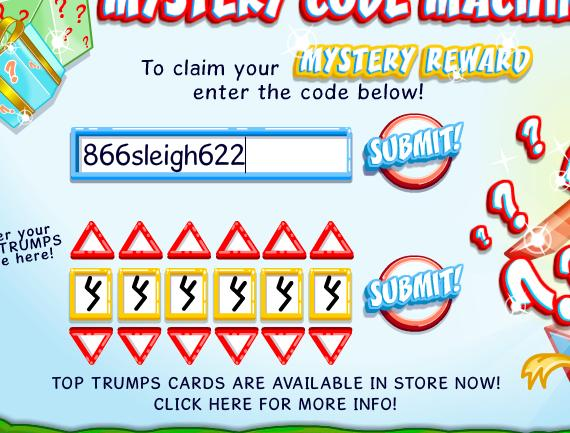You will receive a nest item and 200 mulch!